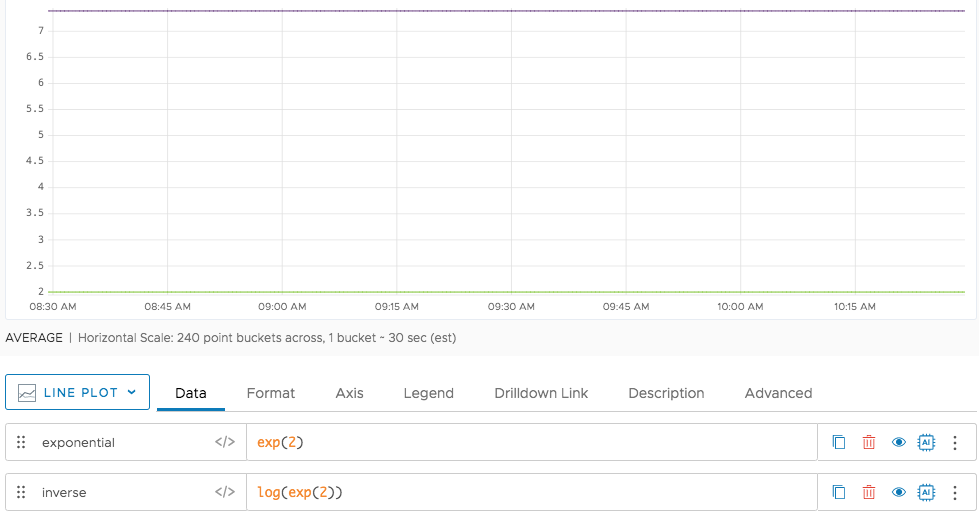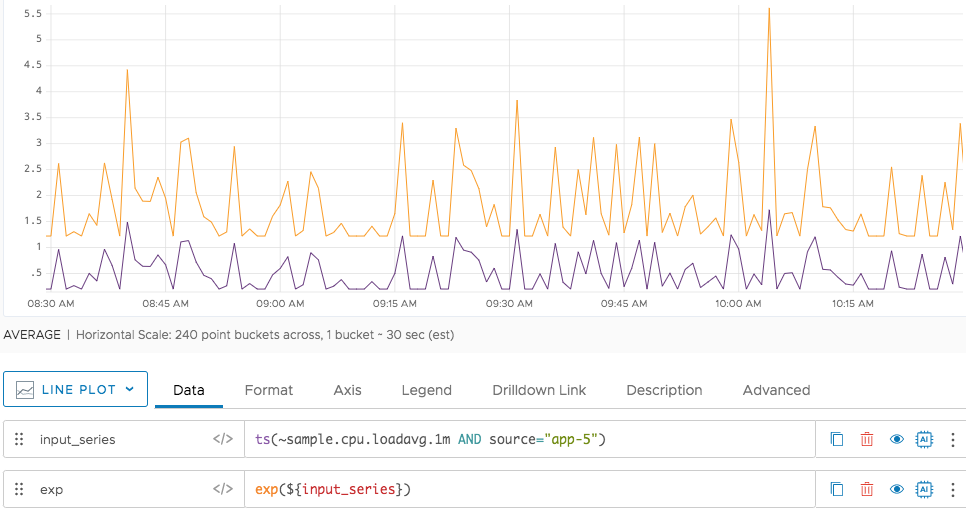Reference to the exp() function

## Summary

``````exp(<tsExpression>)
``````

Returns the natural exponential for each data value described by the expression.

## Parameters

ParameterDescription
tsExpression Expression describing a constant or a time series of values to raise the constant e to.

## Description

The `exp()` function produces a time series in which each data value is equal to e raised to the power specified by the expression.

• If `tsExpression` describes a constant value `N`, then `exp()` returns a continuous series where every data value is `eN`.
• If `tsExpression` describes one or more time series, then `exp()` returns a new time series for each input time series. Each value in a new time series is calculated as `eV`, where `V` is the value of the corresponding point in the input time series.

The inverse of this function is `log()`.

By default, the chart legend displays a rounded version of the result. You can cause the legend to display the result with more precision by holding down the shift key when you hover over the time series.

## Examples

In this example, we see the result (in red) of using `exp(2)` to raise e to the power `2`. We also see the result (in blue) of obtaining the inverse by applying the `log()` function.Here we see the result (in orange) of using `exp()` to obtain the natural exponential of a time series (in purple).